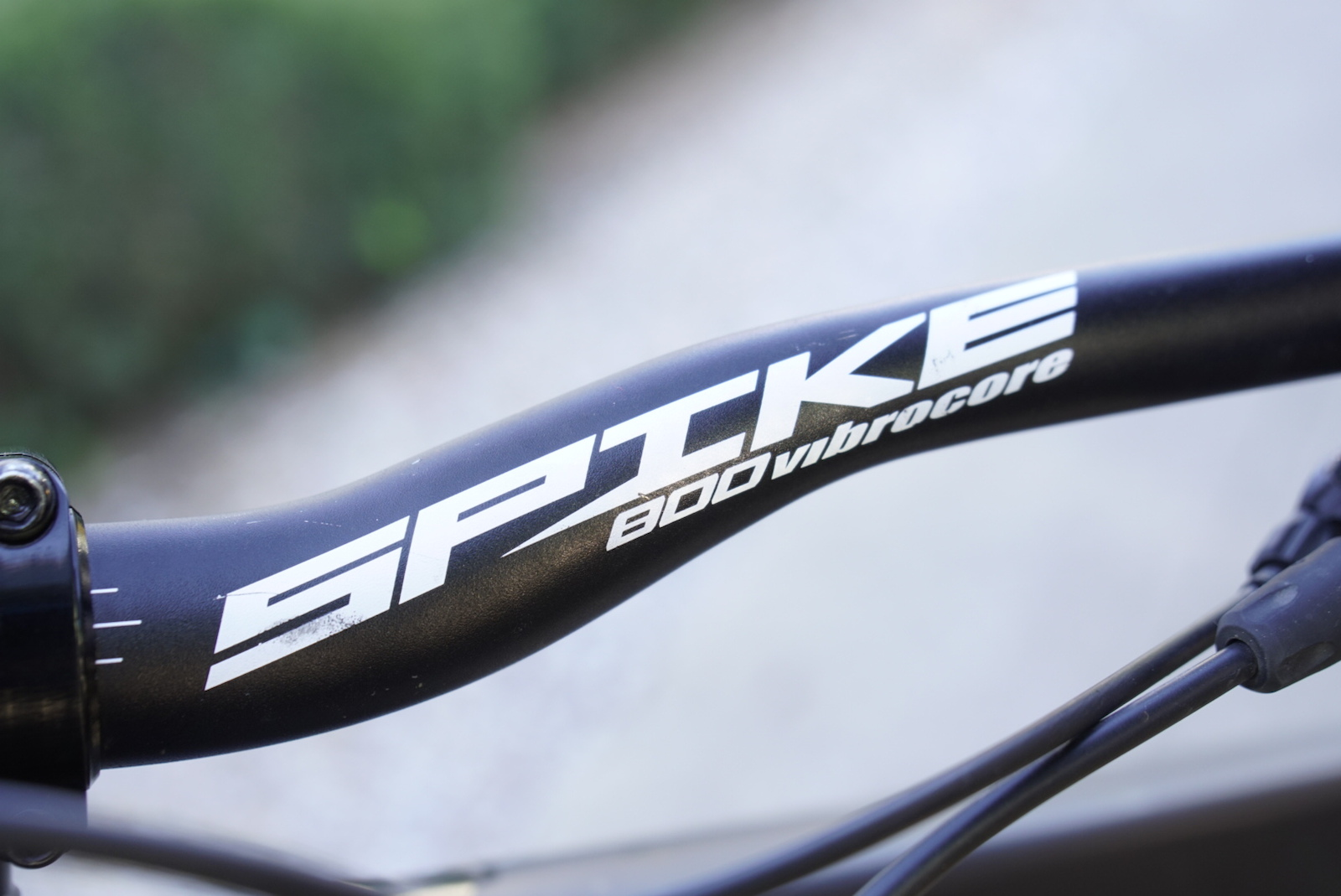=?UTF-8?Q?The_fitnessgram=E2=84=A2_pacer_test_is_a_multi_stage_aerob?= =?UTF-8?Q?ic_capacity_test=2E=2E=2E?=